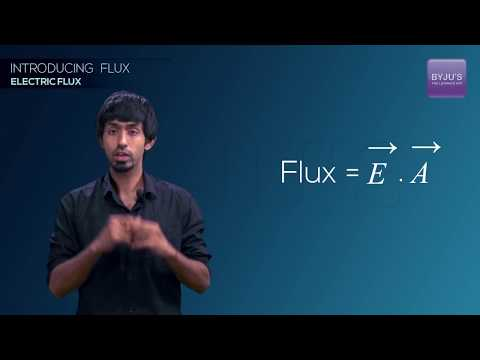Checkout JEE MAINS 2022 Question Paper Analysis : Checkout JEE MAINS 2022 Question Paper Analysis :

# Dimensions of Electric Flux

## Dimensional Formula of Electric Flux

The dimensional formula of electric flux is given by,

[M1 L3 T-3 I-1]

Where,

• M = Mass
• I = Current
• L = Length
• T = Time

### Derivation

Electric Flux (ΦE) = E × S × cos θ . . . . (1)

Where E = magnitude of the electric field and S = Surface Area

The dimensional formula of area (S) = [M0 LT0] . . . . (2)

Since, Electric Field = [Force × Charge-1] . . . (3)

The dimensional formula of,

• Force = m × a = [M1 L1 T-2] . . . (4)
• Charge = current × time = [I1 T1] . . . (5)

On substituting equation (4) and (5) in equation (3) we get,

Electric Field = [M1 L1 T-2] × [I1 T1]-1

∴ The dimensional formula of Electric Field = [M1 L1 T-3 I-1] . . . . (6)

On substituting equation (2) and (6) in equation (1) we get,

⇒ Electric Flux = E × S × cos θ

Or, ΦE = [M1 L1 T-3 I-1] × [M0 LT0] = [M1 L3 T-3 I-1]

Therefore, the electric flux is dimensionally represented as [M1 L3 T-3 I-1].

## Electric Flux⇒ Check Other Dimensional Formulas:

Test your Knowledge on Electric flux